# 日拱一卒，伯克利教你Python核心技术，迭代器和生成器# Topics

## Iterables and Iterators

```python for item in iteralbe: # do something```

for循环可以使用在任何可迭代对象（iterable）中。我们之前描述for循环的时候，说的是它可以用在任何序列上——所有的序列都是可迭代的。但除了序列之外也有其他对象也是可迭代的。实际上，for循环会被解释器转录成类似下面这样的代码：

```python iterator = iter(iterable) try: while True: elem = next(iterator) # do something except StopIteration: pass```

• 首先，`iter`是一个内置函数，它应用在可迭代对象上，生成一个对应的迭代器。迭代器是一个可以在可迭代对象上迭代的对象，它会一直记录下一个被迭代的元素
• `next`函数应用在迭代器上，用来获取序列中的下一个元素
• 当序列中没有下一个元素时，会抛出`StopIteration`异常。在for循环当中，这个异常会被捕获，程序可以继续执行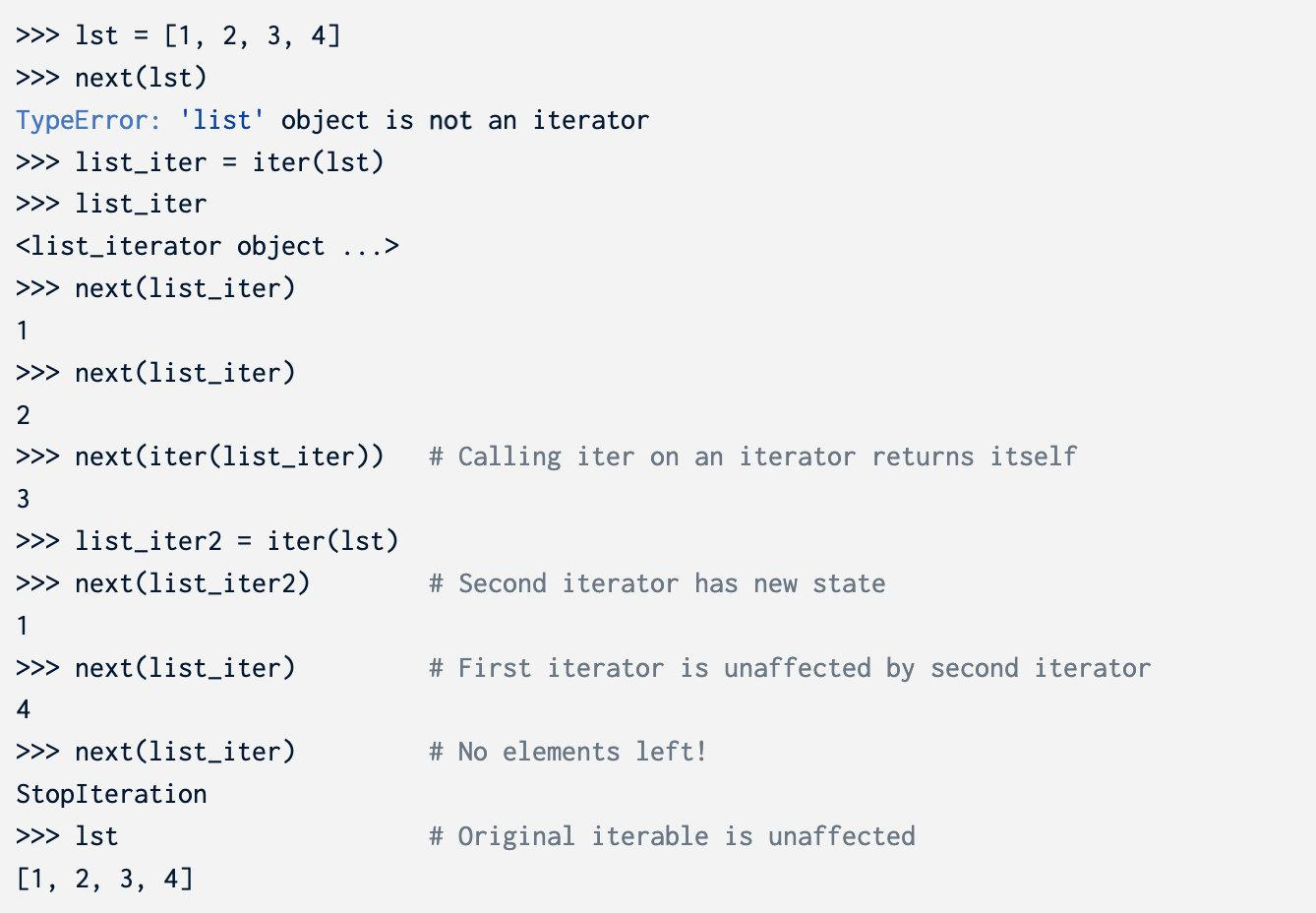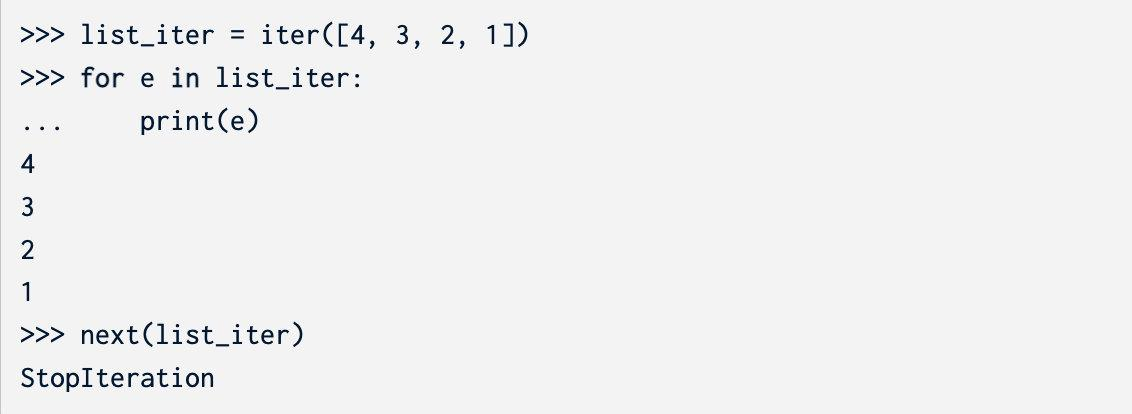### Iterable uses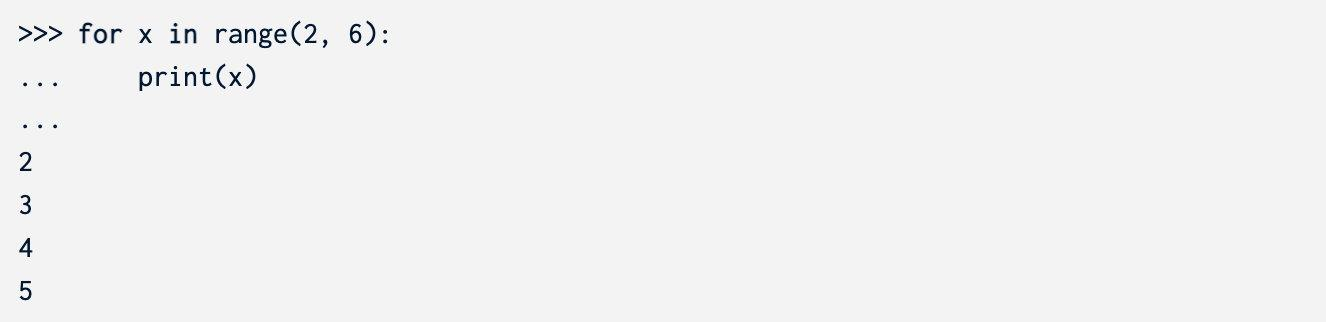• map(f, iterable) - 创建一个迭代器，对iterable中的x，得到`f(x)`
• filter(f, iterable) - 创建一个迭代器，对iterable中的x，得到所有`f(x) == True`的x
• zip(iter1, iter2) - 对`iter1`中的所有x和`iter2`中的所有y，创建一个迭代器，得到所有的`(x, y)`的pair
• reversed(iterable) - 创建一个迭代器可以反向遍历`iterable`
• list(iterable) - 创建一个list，得到`iterable`中的所有元素
• tuple(iterable) - 创建一个tuple，包含`iterable`中的所有元素
• sorted(iterable) - 创建一个排好序的list，包含`iterable`中的所有元素

## Generators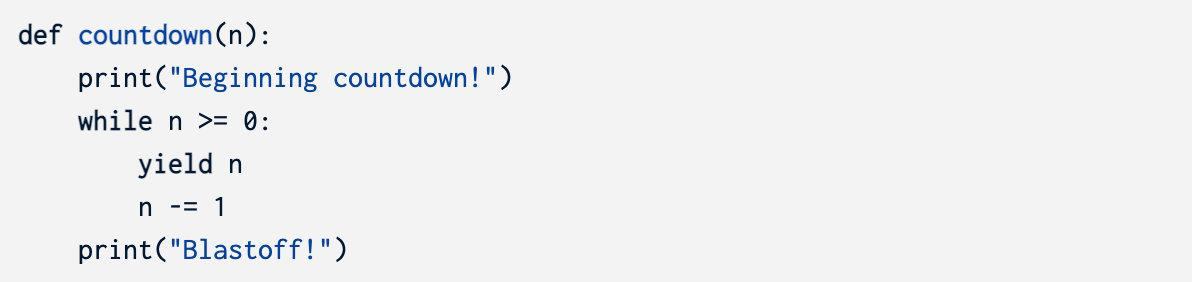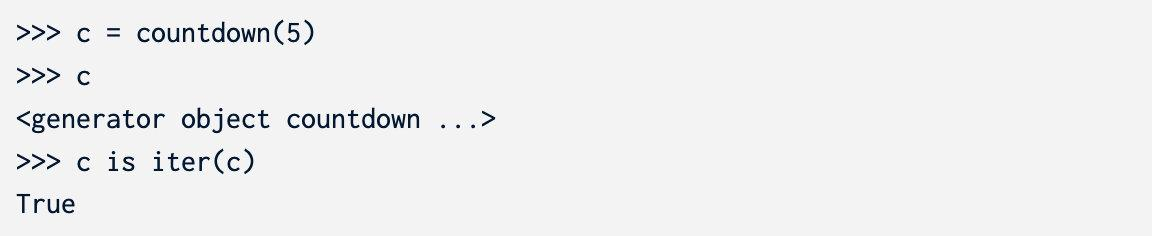`next`被调用的时候，程序会从函数主体的第一行开始执行，一直到遇到`yield`语句。`yield`语句之后的内容将被evaluate并返回。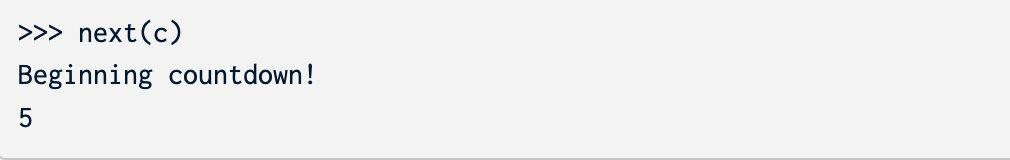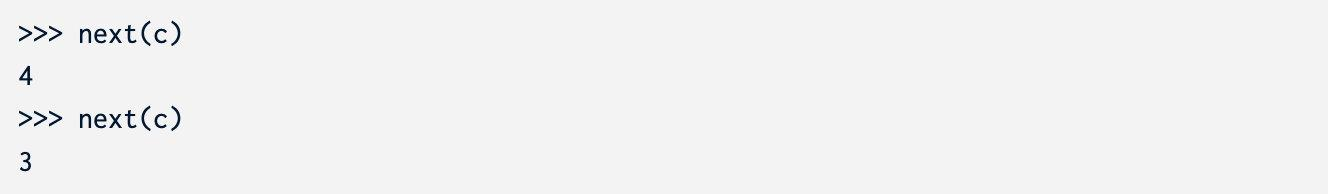`countdown`多次调用会创建不同的生成器，它们拥有专属的状态。通常，生成器不会重启。如果你想要重新得到一个序列，你可以创建另外一个生成器对象。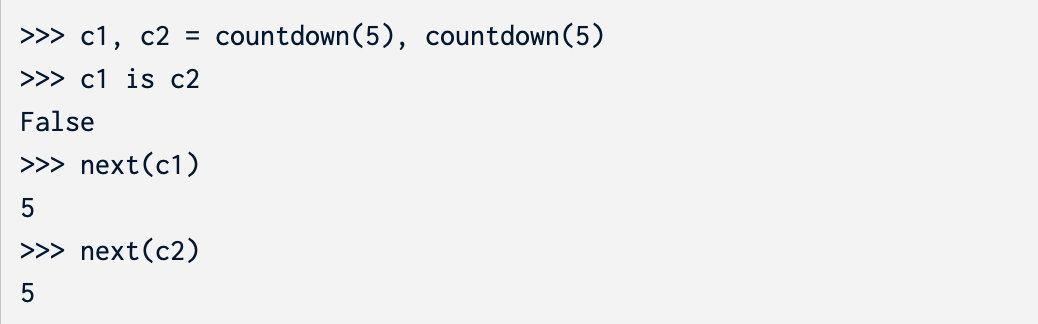• 生成器函数拥有`yield`语句，并且会返回一个生成器对象

• 对生成器对象调用`iter`会得到一个同样的对象，并且不会修改生成器的状态

• 生成器的函数主体不会被执行，直到调用`next`函数。对一个生成器对象调用`next`函数，会运行并且返回序列中的下一个元素。如果序列已经结束了，抛出`StopIteration`异常
• 生成器会记住下一次执行`next`时的状态。

• 第一次调用`next`时：

• 进入函数，运行程序知道遇到`yield`
• 返回`yield`语句中的值，记住`yield`语句的位置
• 持续调用`next`时：

• 从上一次`yield`语句的下一行开始执行，遇见`yield`停止
• 返回`yield`语句中的值，记住当前运行的位置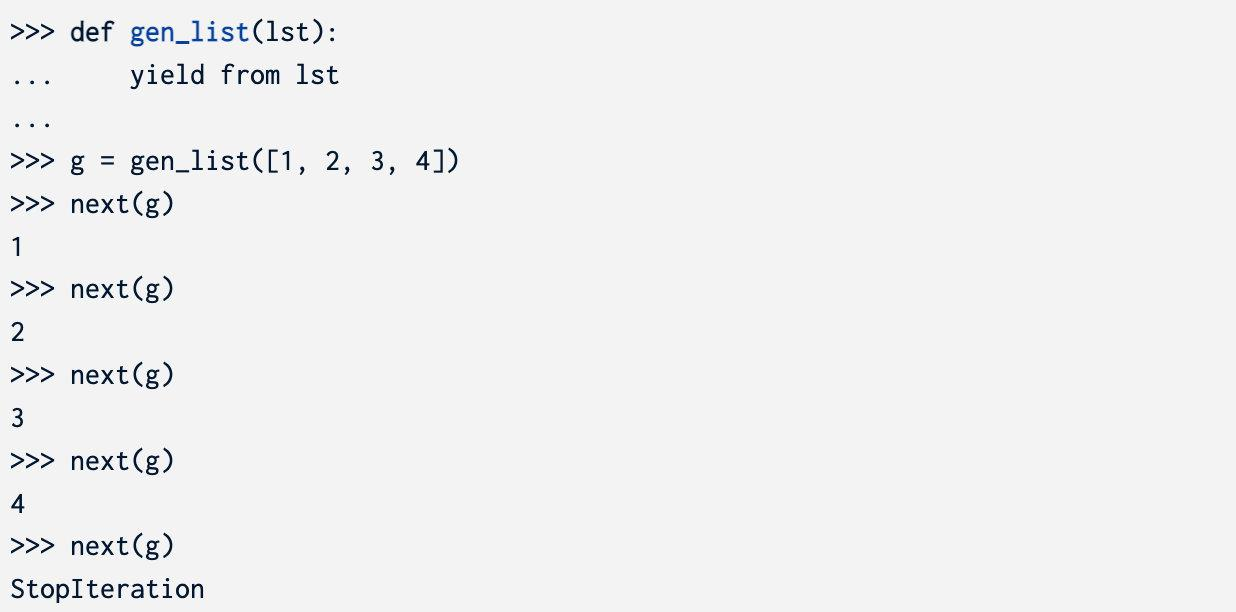# Required Questions

## WWPD

### Q1: WWPD: Iterators

```python

s = [1, 2, 3, 4] t = iter(s) next(s)

next(t)

next(t)

iter(s)

next(iter(s))

next(iter(t))

next(iter(s))

next(iter(t))

next(t)

r = range(6) r_iter = iter(r) next(r_iter)

[x + 1 for x in r]

[x + 1 for x in r_iter]

next(r_iter)

list(range(-2, 4)) # Converts an iterable into a list

map_iter = map(lambda x : x + 10, range(5)) next(map_iter)

next(map_iter)

list(map_iter)

for e in filter(lambda x : x % 2 == 0, range(1000, 1008)): ... print(e) ...

[x + y for x, y in zip([1, 2, 3], [4, 5, 6])]

for e in zip([10, 9, 8], range(3)): ... print(tuple(map(lambda x: x + 2, e))) ...

```

### Q2: WWPD: Generators

```python def gen(): print("Starting here") i = 0 while i < 6: print("Before yield") yield i print("After yield") i += 1

next(gen)

gen

g = gen() g

g == iter(g)

next(g)

next(g)

next(g)

g2 = gen() next(g2)

iter(g2)

next(iter(g))

next(gen())

```

## Coding Practice

### Q3: Scale

```python def scale(s, k): """Yield elements of the iterable s scaled by a number k.

``````>>> s = scale([1, 5, 2], 5)
>>> type(s)
<class 'generator'>
>>> list(s)
[5, 25, 10]

>>> m = scale(naturals(), 2)
>>> [next(m) for _ in range(5)]
[2, 4, 6, 8, 10]
"""
``````

```

#### 答案

```python def scale(s, k): for i in s: yield i * k```

```python def scale(s, k): yield from map(lambda x: x * k, s)```

### Q4: Trap

```python def trap(s, k): """Return a generator that yields the first K values in iterable S, but raises a ValueError exception if any more values are requested.

``````>>> t = trap([3, 2, 1], 2)
>>> next(t)
3
>>> next(t)
2
>>> next(t)
ValueError
>>> list(trap(range(5), 5))
ValueError
>>> t2 = trap(map(abs, reversed(range(-6, -4))), 2)
>>> next(t2)
5
>>> next(t2)
6
>>> next(t2)
ValueError
"""
``````

```

#### 答案

```python def trap(s, k): it = iter(s) for _ in range(k): yield next(it) raise ValueError("no more values")```

# Optional Questions

### Q5: Hailstone

1. 选择一个正整数`n`作为开始
2. 如果`n`是偶数，将它除以2
3. 如果`n`是奇数，将它乘3再加1
4. 重复以上过程，直到`n`等于1

```python def hailstone(n): """ >>> for num in hailstone(10): ... print(num) ... 10 5 16 8 4 2 1 """ "*** YOUR CODE HERE ***"```

#### 答案

```python def hailstone(n): while n != 1: yield n if n % 2 == 0: n //= 2 else: n = n * 3 + 1 yield n```

### Q6: Repeated

```python def repeated(t, k): """Return the first value in iterable T that appears K times in a row.

``````>>> s = [3, 2, 1, 2, 1, 4, 4, 5, 5, 5]
>>> repeated(trap(s, 7), 2)
4
>>> repeated(trap(s, 10), 3)
5
>>> print(repeated([4, None, None, None], 3))
None
"""
assert k > 1
``````

```

#### 答案

```python def repeated(t, k): assert k > 1 "*** YOUR CODE HERE ***" it = iter(t) last, cnt = next(it), 1 for i in it: if i == last: cnt += 1 if cnt == k: return i else: last, cnt = i, 1```

### Q7: Merge

```python def merge(s0, s1): """Yield the elements of strictly increasing iterables s0 and s1, removing repeats. Assume that s0 and s1 have no repeats. s0 or s1 may be infinite sequences.

``````>>> m = merge([0, 2, 4, 6, 8, 10, 12, 14], [0, 3, 6, 9, 12, 15])
>>> type(m)
<class 'generator'>
>>> list(m)
[0, 2, 3, 4, 6, 8, 9, 10, 12, 14, 15]
>>> def big(n):
...    k = 0
...    while True: yield k; k += n
>>> m = merge(big(2), big(3))
>>> [next(m) for _ in range(11)]
[0, 2, 3, 4, 6, 8, 9, 10, 12, 14, 15]
"""
i0, i1 = iter(s0), iter(s1)
e0, e1 = next(i0, None), next(i1, None)
``````

```

#### 答案

```python def merge(s0, s1): i0, i1 = iter(s0), iter(s1) e0, e1 = next(i0, None), next(i1, None) "*** YOUR CODE HERE ***" while e0 is not None or e1 is not None: if e0 is None: yield e1 e1 = next(i1, None) elif e1 is None: yield e0 e0 = next(i0, None) else: if e0 == e1: yield e0 e0, e1 = next(i0, None), next(i1, None) elif e0 < e1: yield e0 e0 = next(i0, None) else: yield e1 e1 = next(i1, None)```

### Q8: Remainder Generator

`remainders_generator`读入一个整数`m`，yield `m`个不同的生成器。第一个生成器是`m`的倍数（对m取模余数为0），第二个生成器是对`m`取模余数为1的生成器，以此类推。

```python def remainders_generator(m): """ Takes in an integer m, and yields m different remainder groups of m.

``````>>> remainders_mod_four = remainders_generator(4)
>>> for rem_group in remainders_mod_four:
...     for _ in range(3):
...         print(next(rem_group))
0
4
8
1
5
9
2
6
10
3
7
11
"""
``````

```

#### 答案

```python def remainders_generator(m): def inner(r): while True: yield r r += m

``````for i in range(m):
yield inner(i)
``````

```

### Q9: Zip Generator

```python def zip_generator(*iterables): """ Takes in any number of iterables and zips them together. Returns a generator that outputs a series of lists, each containing the nth items of each iterable. >>> z = zip_generator([1, 2, 3], [4, 5, 6], [7, 8]) >>> for i in z: ... print(i) ... [1, 4, 7] [2, 5, 8] """ "*** YOUR CODE HERE ***"```

#### 答案

```python def zip_generator(*iterables): itors = [iter(it) for it in iterables] while True: yield [next(it) for it in itors]```